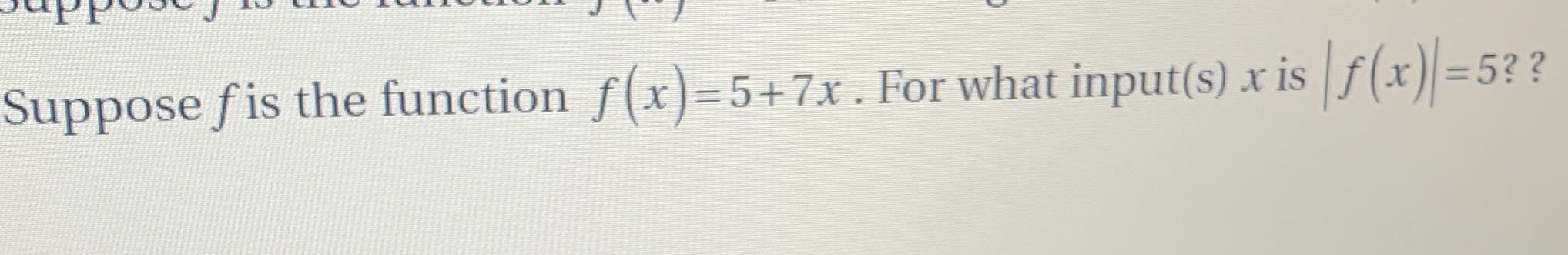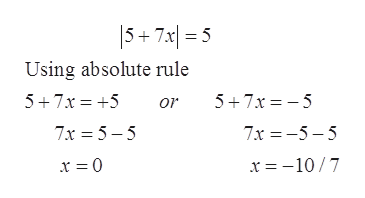# Suppose fis the function f(x)-5+ 7x . For what input(s) x isf(x)=5??

Question
9 views

Help with this problem?help_outlineImage TranscriptioncloseSuppose fis the function f(x)-5+ 7x . For what input(s) x isf(x)=5?? fullscreen
check_circle

Step 1

The given function is f(x) = 5 + 7x

To find the value of x for which |f(x)| = 5.

Step 2

Concept used: Absolute rule

If |x| = a then x = a or x = -a

Step 3

The x can be evaluated ...help_outlineImage Transcriptionclose5+7x 5 Using absolute rule 5+7x 5 5 7x5 or 7x 5-5 7x -5-5 x = -10/7 fullscreen

### Want to see the full answer?

See Solution

#### Want to see this answer and more?

Solutions are written by subject experts who are available 24/7. Questions are typically answered within 1 hour.*

See Solution
*Response times may vary by subject and question.
Tagged in

### Equations and In-equations